# FORECAST

The FORECAST (or FORECAST.LINEAR) function in Excel predicts a future value along a linear trend. The FORECAST.ETS function in Excel predicts a future value using Exponential Triple Smoothing, which takes into account seasonality.

Note: the FORECAST function is an old function. Microsoft Excel recommends using the new FORECAST.LINEAR function which produces the exact same result.

### FORECAST.LINEAR

1. The FORECAST.LINEAR function below predicts a future value along a linear trend.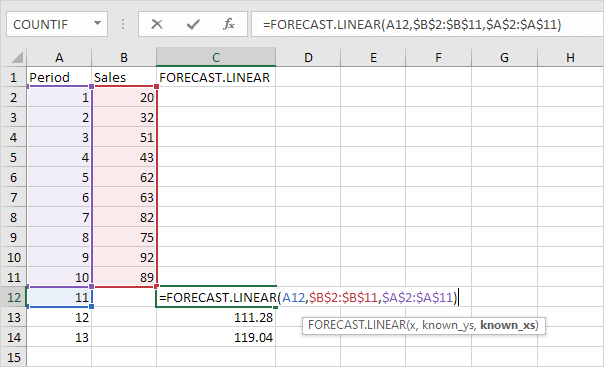Explanation: when we drag the FORECAST.LINEAR function down, the absolute references (\$B\$2:\$B\$11 and \$A\$2:\$A\$11) stay the same, while the relative reference (A12) changes to A13 and A14.

2. Enter the value 89 into cell C11, select the range A1:C14 and insert a scatter plot with straight lines and markers.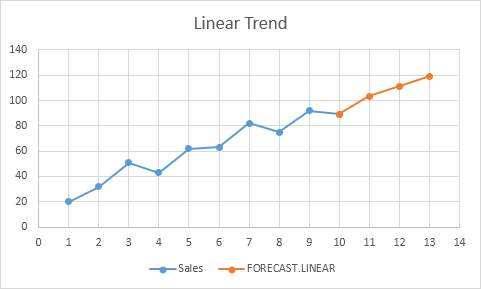Note: when you add a trendline to an Excel chart, Excel can display the equation in a chart. This equation predicts the same future values.

### FORECAST.ETS

The FORECAST.ETS function in Excel 2016 or later is a great function which can detect a seasonal pattern.

1. The FORECAST.ETS function below predicts a future value using Exponential Triple Smoothing.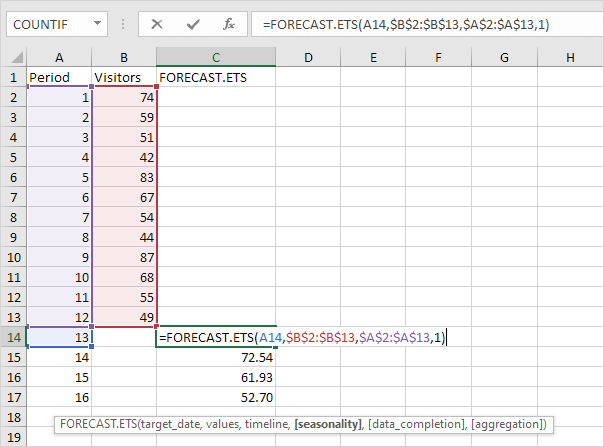Note: the last 3 arguments are optional. The fourth argument indicates the length of the seasonal pattern. The default value of 1 indicates seasonality is detected automatically.

2. Enter the value 49 into cell C13, select the range A1:C17 and insert a scatter plot with straight lines and markers.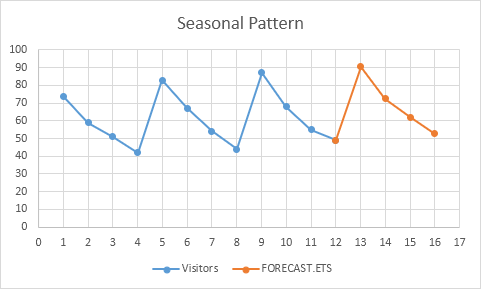3. You can use the FORECAST.ETS.SEASONALITY function to find the length of the seasonal pattern. After seeing the chart, you probably already know the answer.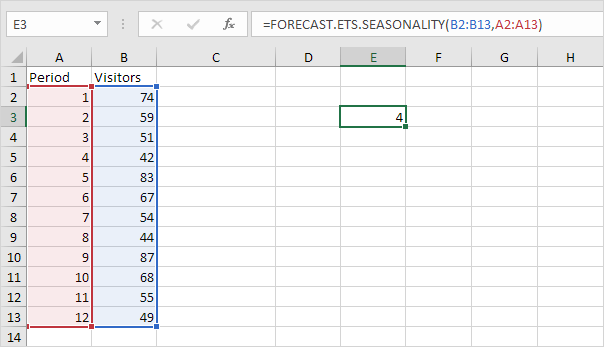Conclusion: in this example, when using the FORECAST.ETS function, you can also use the value 4 for the fourth argument.

### Forecast Sheet

Use the Forecast Sheet tool in Excel 2016 or later to automatically create a visual forecast worksheet.

1. Select the range A1:B13 shown above.

2. On the Data tab, in the Forecast group, click Forecast Sheet.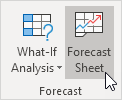Excel launches the dialog box shown below.

3. Specify when the forecast ends, set a confidence interval (95% by default), detect seasonality automatically or manually set the length of the seasonal pattern, etc.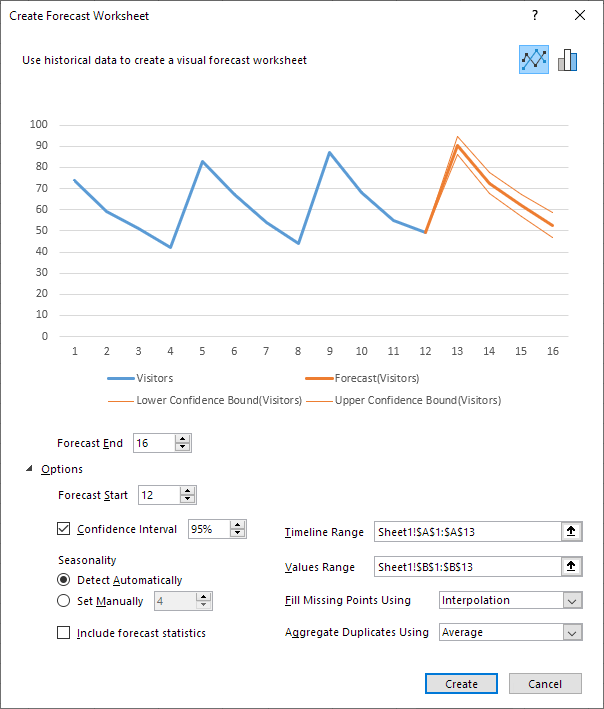4. Click Create.

This tool uses the FORECAST.ETS function and calculates the same future values. The lower and upper confidence bounds are a nice bonus.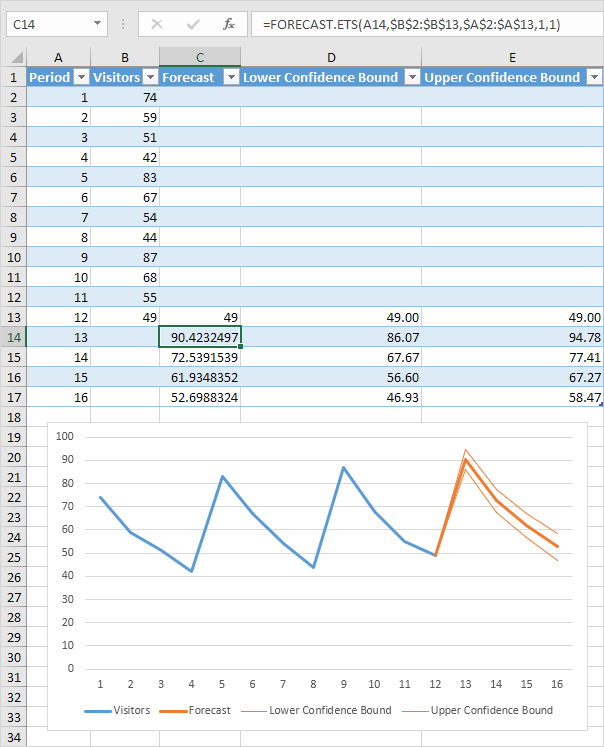Explanation: in period 13, we can be 95% confident that the number of visitors will be between 86 and 94.

Go to Next Chapter: Round